Courses

# Test: Sum And Difference Rule

## 10 Questions MCQ Test Mathematics For JEE | Test: Sum And Difference Rule

Description
This mock test of Test: Sum And Difference Rule for JEE helps you for every JEE entrance exam. This contains 10 Multiple Choice Questions for JEE Test: Sum And Difference Rule (mcq) to study with solutions a complete question bank. The solved questions answers in this Test: Sum And Difference Rule quiz give you a good mix of easy questions and tough questions. JEE students definitely take this Test: Sum And Difference Rule exercise for a better result in the exam. You can find other Test: Sum And Difference Rule extra questions, long questions & short questions for JEE on EduRev as well by searching above.
QUESTION: 1

### The derivation of 40√x - 20

Solution:

dy/dx = 40x½ - 20
dy/dx = 40x½-1 * 20 - 0
= 40/2(x)½
20/(x)1/2

QUESTION: 2

###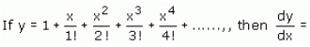​

Solution:

Since the number of terms is finite, just differentiate term by term using the power rule.
dy/dx = 0 + 1 + 2x/2! + 3x2/3! + ... + nxn-1/n!
dy/dx = 1 + x + x2/2! + ... + xn-1/(n -1)!
Compare dy/dx to y and note that the last term of y, which is xn/n!, is not in dy/dx, while all the other terms of y are in dy/dx.
Then dy/dx = y - xn/n!
⇒ dy/dx + xn/n! = y

QUESTION: 3

###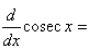Solution:

y = cosecx
= 1/sinx= (sinx)−1
⇒ dy/dx=−(sinx)−2 × d/dx(sinx)
= −cosx/sin2x
= −cosx/sinx ×(1/sinx)
= −cot x cosec x

QUESTION: 4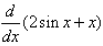Solution:
QUESTION: 5

Find the dervative of 5 sin x - 11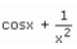Solution:

y = 5 sin x - 11 cosx + 1/x2
dy/dx = 5 cosx - 11(-sinx) + (-2/x3)
= 5cosx + 11sinx - 2/x3

QUESTION: 6

The dervative of the function 3x2 - x is :

Solution:

dy/dx = 3x2-x
dy/dx = 3(2)x(2-1) - 1x(1-1)
= 6x-1

QUESTION: 7

The derivate of the function sin x + cos x is :

Solution:

Y=sinx+cosx
dy/dx=cosx-sinx

QUESTION: 8

The function, f(x) =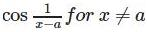and f(a) = 0, is

Solution:
QUESTION: 9

The derivative of the function f(x) = 2x2+3x-3 at x = – 2 is:​

Solution:

2x2 + 3x - 3 =0
dy/dx = 4x + 3
At x = -2
4(-2) + 3 = -5

QUESTION: 10

The derivate of x3 - 20x at x = 10 is :

Solution:

Y=x3-20x
dy/dx=3x2-20
(dy/dx) at x=10 is
=3×100-20
=280